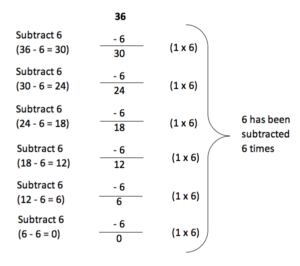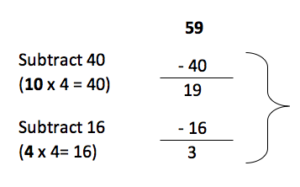What is the chunking method? The chunking method is a way of dividing larger numbers by repeatedly subtracting the number you are dividing by.

The chunking method is helpful because it breaks down the question by using the multiplication and division facts that a child is most confident with.

## When will children learn the chunking method?

When children first learn to divide, they may be introduced to the chunking method as it encourages them to use the multiplication skills they have learnt from their times tables.

Around Years 5 and 6, when children are happy using the chunking method, teachers will get them to try using faster methods of division, such as short division (called the ‘bus stop method’).

## How to use the chunking method

Question: 36 ÷ 6Children should subtract the number 6 from 36 and write the answer down underneath a line. Then they should keep repeating this process until the number 6 can no longer be subtracted.

The final step is to work out how many times 6 was subtracted, which was 6 times.

As we are left with 0, there is no remainder.

Therefore, 36 ÷ 6 = 6.

Children should subtract the number 6 from 36 and write the answer down underneath a line. Then they should keep repeating this process until the number 6 can no longer be subtracted.

The final step is to work out how many times 6 was subtracted, which was 6 times.

As we are left with 0, there is no remainder.

Therefore, 36 ÷ 6 = 6.

Using the chunking method will require that children are confident in their multiplication and division facts. For example, they should understand that as 36 ÷ 6 = 6, then 6 x 6 = 36. If your child is struggling to use the chunking method, it might be useful to go back over their times tables and make sure that they are confident with the relationship between division and multiplication.

Sometimes, children will need to use the chunking method for questions where there will be a remainder (a number left over). For example:

Question: 59 ÷ 4 (or how many 4s go into 59)Then you work out how many 4s have been subtracted (10 + 4 = 14).

14 lots of 4, with 3 left over.

59 ÷ 4 = 14 r3

In the example above, the calculation ’10 x 4’ is used because many children will be confident with their 10 times table. It is also helpful to subtract a big number to start with as it speeds up the division.

## Why is the chunking method useful?

Using the chunking method makes dividing larger numbers easier for children because it allows them to work out several smaller calculations to get the final answer. It also means that children are having to consider how multiplication and division are related, their times table knowledge should be able to help them with this.

## How does Learning Street help children with the chunking method?

The Learning Street courses will introduce the idea of chunking to the child and then have practice questions and exercises. This ensures that the basic knowledge is understood before there is further extension and development for the child.

Tests could include SATs, competitive 11 Plus tests or selective Independent school exams.

## Our Courses

Click through to review the courses we have available

##### Get Started

Learning Street structured courses make home study easier and more successful. There's no need to wonder which books to buy or what to do next. Everything is planned for you.

• Proven to deliver excellent results
• Used by families, private tutors and schools
• Fully planned and structured